Jia, X., Zhao, J., and Cai, Y. (2015). "Radio frequency vacuum drying of timber: Mathematical model and numerical analysis," BioRes. 10(3), 5440-5459.

#### Abstract

The mass and heat transfer mechanisms during radio frequency/vacuum (RF/V) drying of square-edged timber were analyzed and discussed in detail. Based on the mass and heat transfer theory of porous materials, a one-dimensional mathematical model was developed from conservation equations. Compared with conventional models, it has the following advantages: (1) Each independent variable has a separate governing equation and is solved independently by converting the partial differential equation into a difference equation with the finite volume method; and (2) The calculated data from different parts of the wood specimen are displayed in the evolution curves because the specimen is divided into several units along fiber direction. Therefore, the change law of the parameters can be better described. The software Matlab, which has the advantages of fast calculation speed and high precision, was used for programming and calculations. In addition, the square-edged timber Sugi specimen was dried in a laboratory RF/V dryer, and the total average moisture content (MC) and local temperature were monitored as a function of time. By analyzing the calculated and experimental results, it can be concluded that most of the important phenomena observed during RF/V drying can be adequately described by this model.

#### Full Article

Radio Frequency Vacuum Drying of Timber: Mathematical Model and Numerical Analysis

Xiaoran Jia,a,b Jingyao Zhao,a and Yingchun Cai a,*

The mass and heat transfer mechanisms during radio frequency/vacuum (RF/V) drying of square-edged timber were analyzed and discussed in detail. Based on the mass and heat transfer theory of porous materials, a one-dimensional mathematical model was developed from conservation equations. Compared with conventional models, it has the following advantages: (1) Each independent variable has a separate governing equation and is solved independently by converting the partial differential equation into a difference equation with the finite volume method; and (2) The calculated data from different parts of the wood specimen are displayed in the evolution curves because the specimen is divided into several units along fiber direction. Therefore, the change law of the parameters can be better described. The software Matlab, which has the advantages of fast calculation speed and high precision, was used for programming and calculations. In addition, the square-edged timber Sugi specimen was dried in a laboratory RF/V dryer, and the total average moisture content (MC) and local temperature were monitored as a function of time. By analyzing the calculated and experimental results, it can be concluded that most of the important phenomena observed during RF/V drying can be adequately described by this model.

Keywords: RF/V drying; Mathematical model; Transport mechanism; Difference equation; Finite volume method

Contact information: a: Key Laboratory of Bio-based Material Science and Technology of Ministry of Education, Northeast Forestry University, 150040, Harbin, China; b: Heilongjiang Institute of Wood Science,150032, Harbin, China; *Corresponding author: caiyingchunnefu@163.com

INTRODUCTION

The RF/V drying of wood is a typically unsteady and irreversible process with a mass and heat coupling effect, which also includes a water phase change and the thermal mass transfer effect of a multiphase, multicomponent mixture. This process is influenced by many factors such as the properties of the wood, heat flux of the RF, environmental conditions, etc. It is difficult to monitor the MC and the temperature of the wood during the drying process. Thus, developing a proper mathematical model is beneficial for assisting with the design of the drying parameters.

There have been more extensive reviews (Rosen 1987; Kamke and Vanek 1992) of models of wood drying developed through the years. A classification of drying models for porous solids and a good description of all possible transfer mechanisms can be found in Waananen et al. (1993). A good description of how to develop a relevant model for wood drying is presented by Perre (1999). Most of the models developed describe convective wood drying. A limited number of papers exist in modeling vacuum drying with dielectric drying (Chen and Schmidt 1990; Turner and Jolly 1991; Constant et al. 1996; Chen and Schmidt 1997; Defo et al. 1999; Li et al. 2008). These cases are different from RF/V drying; only a few researchers have obtained research results in this field (Kounmoutsakos and Avranmidis 1989; Kanagawa 1989; Avranmidis and Liu 1994; Kounmoutsakos et al. 2001a,b).

The purpose of this paper is to develop a new mathematical model of RF/V drying that can simulate the real drying process. The most important significance is to grasp the change law of the MC, temperature, and other parameters during the drying process constantly and accurately. Meanwhile, a successful model can lay thefoundation for the automatic control of wood drying and provide essential information for improving drying quality. The new model is different from the others in that (1) It avoids combining all the independent variables that are coupled with each other into a system of two partial differential equations (Chen and Schmidt 1990; Turner and Jolly 1991; Couture et al. 1995), which makes the calculation process too complicated. Each independent variable has its independent governing equation and can be solved independently by converting the partial differential equation into difference equation with the finite volume method; (2) The MC and temperature evolution curves from different parts of the specimen can be displayed, which reflect the differences in MC and temperature distributions in different positions of the wood clearly; and (3) The calculation method and processing of the model (especially the difference equations) are given. Therefore, it is convenient and effective to use the Matlab software for programming and calculations.

EXPERIMENTAL

Mass and Heat Transfer Mechanisms in Wood during RF/V Drying

Mass transfer mechanism

The moisture in wood is in two different forms: free water that occurs when the MC is above the fiber saturation point (FSP), and bound water that occurs when the MC is either above or below the FSP. For purposes of simplification, the presence of an adsorbed water layer is ignored above the FSP. Because of these two forms, there are two different periods of the drying process that follows the two different drying mechanisms described below. The schematic diagram for moisture transfer in wood is shown in Fig. (1).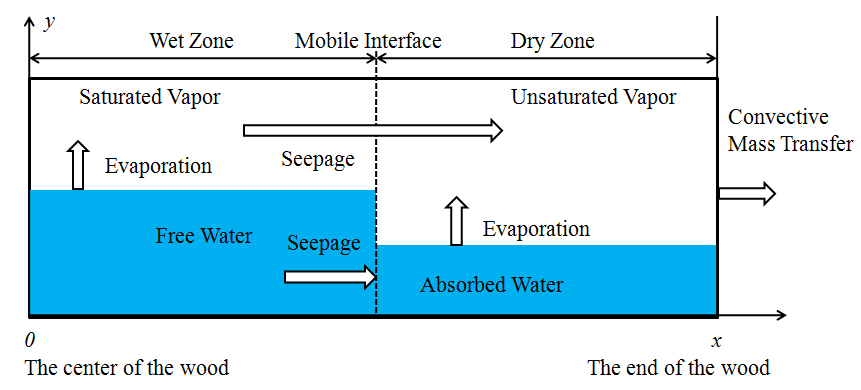Fig. 1. Mass transfer in wood

MC above FSP (wet zone): Under continuous RF heating, the internal temperature of the wood increases rapidly during the initial drying period; meanwhile, most of the liquid phase water (free water) in the pores of the wood evaporates into vapor (arrow↑in Fig. 1). The capillary tension is considered to be the driving force of liquid phase water in convective wood drying (Perre and Turner 1997), but the concave surface of free water in the capillary is damaged when the temperature reached the boiling point; thus the capillary tension is negligible. The vapor pressure increases gradually because of the continuous evaporation of free water until the vapor is saturated, corresponding to the local temperature. The ambient pressure is low (10 to 20 kPa) because of the presence of vacuum, and it remains unchanged under the action of the vacuum pump. Thus the internal mass transfer is strongly enhanced by the pressure difference, which is created by internal water evaporation. According to the relationship between the temperature, saturated vapor pressure, and saturated vapor density above FSP (Cai and Hayashi 2001a,b), the vapor maintains phase equilibrium at all times, and the ambient vapor density corresponding to the ambient pressure is also low, thereby forming large gradients of temperature, pressure, and density that exhibit higher levels inside and lower levels outside. Because of the lower resistance, the ratio of the vapor bulk flow along the fiberdirection is much higher than that of the other direction (Couture et al. 1995). Thus, it can be concluded that the free water and vapor are transferred to the surroundings by the total pressure gradient through the large capillary path along the fiber direction (arrow→in Fig.1). Most moisture is mainly removed by evaporation, and the intrinsic and relative permeability of each of the different phases are the most important factors under consideration in a RF/V drying model (Kounmoutsakos et al. 2001a).

MC below FSP (dry zone): The bound water is only present in the tiny capillaries on the cell wall. The same as in the wet zone, the concave surface of bound water in the capillary was damaged when the temperature reached the boiling point, so the capillary tension was negligible. The diffusion of bound water is considered to be the predominant mass transfer mechanism (Kounmoutsakos et al. 2001a), and the magnitude of the diffusion is low compared with evaporation. Therefore, the diffusion of bound water was negligible. The bound water could only evaporate into vapor, which moved into the cell cavity and was transferred to the surroundings by the total pressure gradient through the same path as the free water.

Though the specimen wood is in the vacuum environment, there is still a gas flow around the surface of the wood because of the action of the vacuum pump keeping the vacuum degree of the kiln. Therefore, a convective mass transfer is created between the surface of the wood (arrow→in Fig. 1) and the environment.

Heat transfer mechanism

The schematic diagram for heat transfer in wood is shown in Fig. 2. RF heating is a type of dielectric heating that has selectivity; that is, areas with higher MC absorb more power, which means that the power intensity of these areas is higher. When the wood interior is heated by the RF generator (arrow↑in Fig. 2), the energy is transferred from the wood interior to the surroundings because of the effect of heat conduction (arrow→in Fig.2). At the same time, part of the energy is consumed by the bulk flow of free water and vapor, and another part is consumed by evaporation (arrow↓in Fig. 2). A convection heat transfer exists between the surface of the wood and the environment (arrow→in Fig. 2), which results in the lower temperature at the surface part, therefore a temperature gradient is formed such that the internal temperature is higher than the external temperature.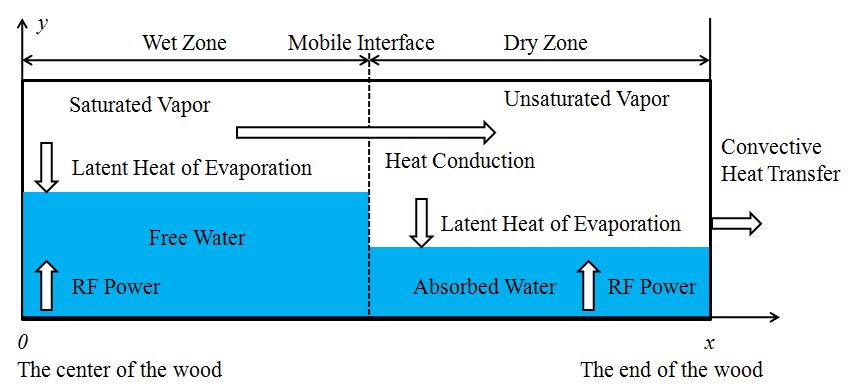Fig. 2. Heat transfer in wood

Assumptions

According to the analysis of the mass and heat transfer mechanisms above, in order to facilitate the model’s construction and solution, the structure of the wood and the physical drying process were simplified. The main assumptions used to formulate the model are shown as follows: 1) The actual complex timber structure is regarded as a network system composed of many large and tiny capillaries; and the liquid phase water present in the large capillaries is called free water. The vapor in the large capillaries maintains a state of saturation at all times. The liquid phase water present in the tiny capillaries is called bound water, and the liquid phase and gas phase maintain phase equilibrium at all times; 2) The bound water cannot be transferred in the liquid state below the FSP by bulk flow or diffusion, only by evaporation; 3) All phases in the wood are in the local thermodynamic equilibrium state, i.e. the temperature of all phases is the same; 4) Though there is the phenomenon of drying shrinkage when the bound water is transferred during the drying process, assuming the solid skeleton is rigid, the size and the internal structure of the wood are unchanged; 5) It is considered that the mass and heat are transferred along the one-dimensional direction (fiber direction, x axis); 6) The energy loss of heat conduction, convective heat transfer, and phase change heat transfer are negligible; 7) The initial MC and temperature of the wood are uniform throughout the specimen; 8) The ambient conditions are stable; and 9) The vapor is regarded as an ideal gas.

Governing Equations

Diffusion and seepage of water

The transfer of liquid phase water in porous materials comes mainly in two forms: diffusion and seepage. Diffusion is usually described by Fick’s law (Siau 1984). If internal evaporation is neglected, then liquid water can only be transferred along the one-dimensional direction (x axis) (Eq. 1),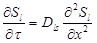(1)

where Sis the liquid phase water saturation, Dls is the mass diffusivity (m2/s), τ is time (s), and x is space (m).

The relationship between the MC and the liquid phase water saturation is described by Eq. 2,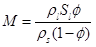(2)

where ρis the liquid phase water density (kg/m3), ρis the wood substantial density (kg/m3), and Φ is the wood porosity.

Seepage is usually described by Darcy’s law (Siau 1995), as is given in Eq. 3,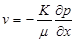(3)

where K is the intrinsic permeability (m2/ Pa·s), μ is the viscosity (Pa·s), and v is the velocity (m/s).

The seepage of liquid phase water follows the principle of mass conservation, as is given in Eq. (4),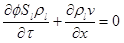(4)

where it is assumed that the relationship between the capillary pressure and the liquid phase water saturation is directly proportional. Substitution of Eq. 3 into Eq. 4 leads to Eq. 5: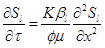. (5)

The form of Eqs. 1 and 5 are basically the same. Therefore, the method used in this paper was to use the diffusion equation to describe the seepage process by unifying Fick’s law and Darcy’s law, i.e. l/Φμ is equal to Kls (effective permeability), where βis the proportionality coefficient.

Mass balance:

Above FSP: The mass conservation equation of free water is given in Eq. 6,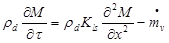(6)

where ρd is the absolute dry density of wood (kg/m3) and is the volume evaporation rate (kg/m3·s). The variation in the mass of free water per unit time per unit volume (first term on the left-hand side) is equal to the variation in mass of free water that is transferred in or out of the unit (first term on the right-hand side) and the mass of the free water that is lost in evaporation (second term on the right-hand side).

The mass conservation equation of vapor is given in Eq. 7,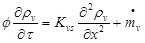(7)

which describes that the variation in mass of vapor per unit time per unit volume (first term in the left hand side) is equal to the variation in mass of vapor that is transferred in or out of the unit in the form bulk flow (first term on the right-hand side) and the mass of the vapor formed by evaporation of the free water (second term on the right-hand side). According to Eq. (1), the saturated vapor density is determined by the local temperature above FSP (Cai and Hayashi 2002). Therefore, Eq. 7 is the governing equation of vapor density below FSP. The governing equation of volume evaporation rate can be gained by converting Eq. 7 into Eq. 8,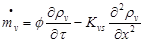(8)

where Kvs is the effective permeability of vapor (m2/s) and ρis vapor density (kg/m3).

Below FSP: According to Eq. 2, the bound water could only evaporate, therefore the mass conservation equation of the bound water is given in Eq. 9,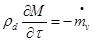(9)

which describes that the variation in mass per unit time per unit volume is equal to the mass of the free water that is lost by evaporation.

Thermodynamic equations:

Above FSP: According to Eq. (1), the vapor pressure and vapor density can be calculated by checking the “Saturated water and saturated steam thermodynamic properties table.”

Below FSP: The vapor is unsaturated vapor (superheated vapor), and the unsaturated vapor pressure can be calculated by local relative humidity and the saturated vapor pressure corresponding to the local temperature (Cai and Hayashi 2002), as is given in Eq. 10: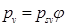. (10)

According to Eq. 9, the vapor density can be calculated by the Clausius-Clapeyron relation, as is given in Eq. 11: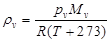, (11)

where φ is the relative humidity, R is the universal gas constant, and Mis the molecular weight of water (kg/mol).

Energy balance:

Above FSP: The energy conservation equation is given in Eq. 12: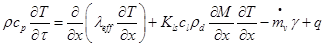(12)

where cis the total heat capacity (J/kg·K), λeff is the effective thermal conductivity (W/m·K), cl is the heat capacity of free water (J/kg·K), is the RF power density (J/m3·s), and γ is the latent heat of vaporization (J/kg). The variation in energy of the wood per unit time per unit volume (the term in the left hand side) is equal to the variation in energy transferred in or out of the unit in the form of conduction (first term in the right hand side), the increase in the energy carried by the free water (second term in the right hand side), the decrease in energy consumed for phase change of free water to vapor (third term in the right hand side), and the increase in energy caused by RF heating (fourth term in the right hand side). The energy carried by vapor is negligible because of its low magnitude compared with the others.

Below FSP: The energy conservation calculation is given in Eq. (13). It is the same as Eq. (12) except for lacking the term that presents the decrease in the energy consumed by the phase change of free water into vapor: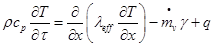(13)

RF power

RF heating is a type of dielectric heating for which the dielectric loss factor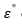relates to the MC (Zhou and Avramidis 1999);decreases with decreasing MC during the drying process. Assuming the relationship between the dielectric loss factor and MC is directly proportional, the electric energy absorbed by the dielectric (wet wood) per unit volume (power density) is given in Eq. 14,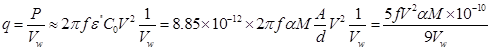(14)

where P is the electric power, C0 is the capacity plate antenna (F), Ais the area of the plate electrode (m2), is the distance between the two plate electrodes (m), is the electric field frequency (Hz), is the plate voltage (V), α is the proportional constant, and Vw is the volume of the specimen wood (m3).

Definite Condition

In order to solve the equations above, initial conditions (I.C.) and boundary conditions (B.C.) are required for each equation. These are as follows:

I.C.

At τ=0, according to Eq. (7), MC and temperature are set equal to the known initial MC and initial temperature of the specimen wood as it enters the kiln.

B.C.

1. MC

At x=0, the center of the wood.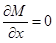(15)

At x= L/2, the end of the wood.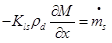(16)

Equation (16) shows that the mass of the water transferred from the wood interior to the surface per unit time per unit surface area is equal to the mass of the liquid phase water that evaporates. The quantityis the surface evaporation rate (kg/m2·s).

The convective mass transfer equation is given in Eq. 17,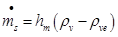(17)

where ρve is the ambient vapor density (kg/m3) and hm is the convective mass transfer coefficient (m/s).

2. Vapor density

At x= L/2, the end of the wood.

Equation 18 shows that the mass of the vapor transferred from the wood interior to the surface per unit time per unit surface area is equal to the decrease in the mass of the vapor caused by convective mass transfer: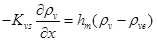, (18)

3. Volume evaporation rate

The volume evaporation rate doesn’t need B.C itself; however, according to Eq. 8, it is determined by the vapor density; thus at x=L/2, the B.C is the same as Eq. (18).

4. Temperature

At x=0, the center of the wood.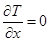(19)

At x= L/2, the end of the wood.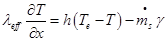(20)

Equation (20) shows that the energy transferred to the surface of the wood in the form of heat conduction per unit time per unit surface area is equal to the decrease in energy caused by the convection heat transfer (first term on the right hand side) and energy consumed due to the evaporation of the liquid phase water (second term on the right hand side). The quantity h is the convective heat transfer coefficient (W/m2·K).

Difference Equations

The governing equations of mass and heat transfer are complicated nonlinear partial differential equations. In order to easily calculate the numerical solutions with Matlab, the partial differential equations need to be transformed into difference equations. The basic idea is to substitute the set of values that contain a series of a finite number of discrete points (nodes) for the continuous physical quantity in the time and space coordinates, and then calculate the differenceequations by the iterative method (Chen and Schmidt 1997; Yu 2010; Wang 2012).

In this paper, the governing equations and corresponding B.C. were discretized in the range of calculation using the finite volume method, and an explicit scheme method was selected for time and space integrations. As is shown in Fig. 3, the specimen is divided into JZ (JZ=10) elements from the center to the end, the length of each element is △(space step)the nodes j＝1,2，．．．．JZ. The mobile interface is in the unit j=JZIN, in which the MC is equal to the FSP.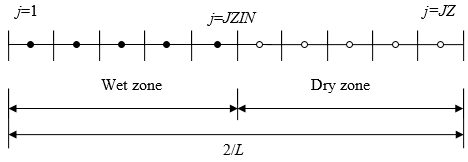Fig. 3. Elements and nodes of the discrete area

Difference equations of MC

1. above FSP

For j=1, the difference equation was obtained from the B.C. in Eq. (15), and the schematic diagram is shown in Fig. (4a).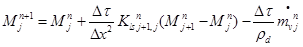(21)

For 1<j<JZIN, the difference equation was obtained from Eq. 6, and the schematic diagram is shown in Fig. 4b.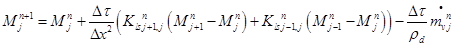(22)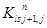and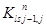are harmonic mean items.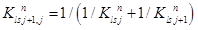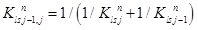For j=JZIN, if JZIN=JZ, namely MJZMFSP, the difference equation was obtained from the B.C. in Eq. (16), and the schematic diagram is shown in Fig. (4c).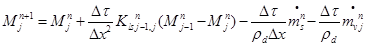(23)

For j=JZIN, if JZIN<JZ, the difference equation is the same as in Eq. (22).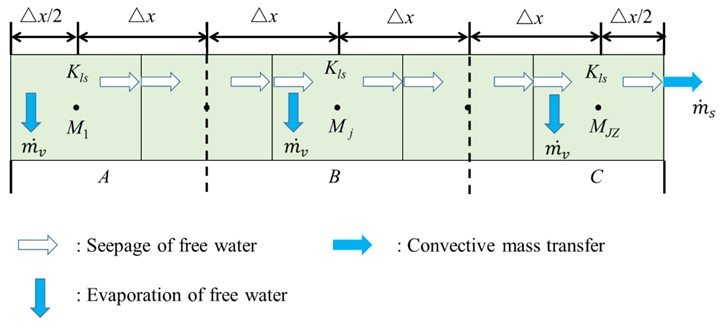Fig. 4. Difference equation of mass transfer above FSP

2. below FSP

For j=JZIN+1, the difference equation is given in Eq. (24), and the schematic diagram is shown in Fig. (5a).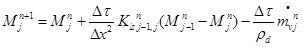(24)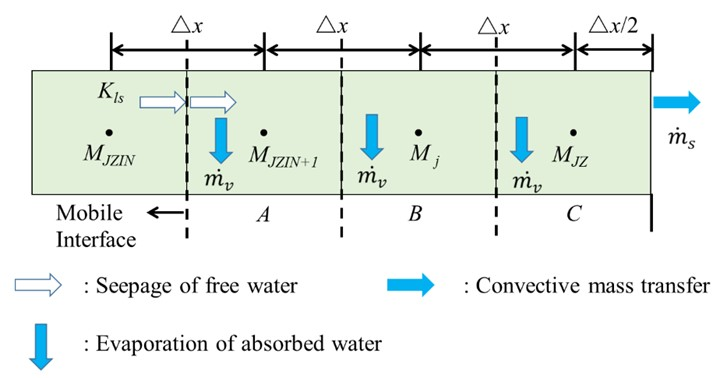Fig. 5. Difference equation of mass transfer below FSP

For JZIN+1<j<JZ, the difference equation was obtained from Eq. (9), and the schematic diagram is shown in Fig. (5b).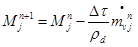(25)

For j=JZ, namely MJZ<MFSP, the difference equation was obtained from the B.C. in Eq. (16), and the schematic diagram is shown in Fig. (5c).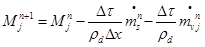(26)

Difference equations of vapor density

1. below FSP

For JZIN<j<JZ, the difference equation was obtained from Eq. (7), and the schematic diagram is shown in Fig. (6a).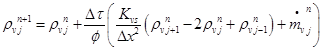(27)

For j=JZ, the difference equation can be obtained from the B.C. in Eq. (18), and the schematic diagram is shown in Fig. (6b).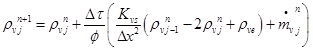(28)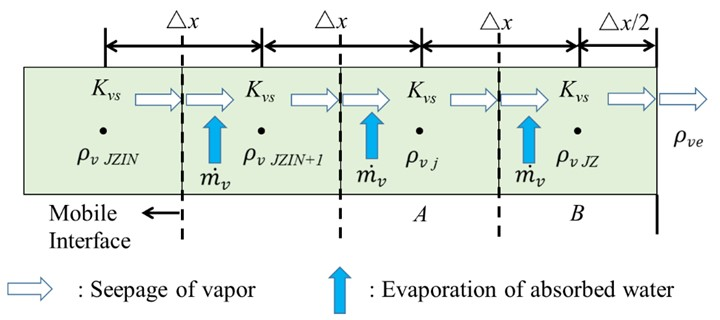Fig. 6. Difference equation of vapor transfer

Difference equations of temperature

1. above FSP

For j=1, the difference equation can be obtained from the B.C. in Eq. 19, and the schematic diagram is shown in Fig. 7a.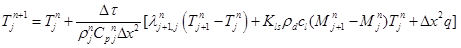(29)

For 1<j<JZIN, the difference equation can be obtained from Eq. 12, and the schematic diagram is shown in Fig. 7b.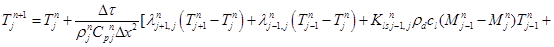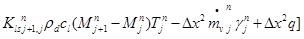(30)

For j=JZIN, if JZIN=JZ, the difference equation can be obtained from the B.C. in Eq. 20, and the schematic diagram is shown in Fig. 7c.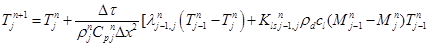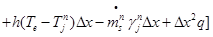(31)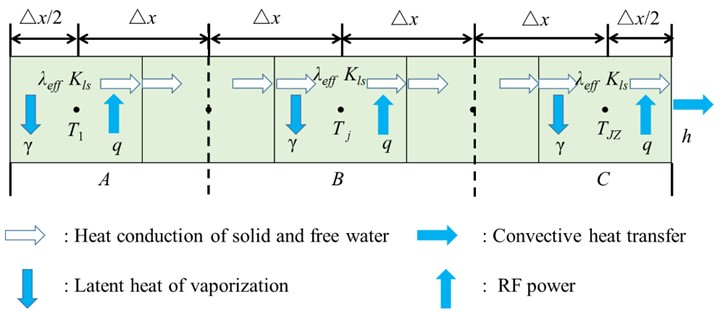Fig. 7. Difference equation of heat transfer above FSP

2. below FSP

For j=JZIN+1, the difference equation is given as Eq. 32, and the schematic diagram is shown in Fig. 8a.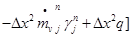(32)

For JZIN+1<j<JZ, the difference equation can be obtained from Eq. 13, and the schematic diagram is shown in Fig. 8b.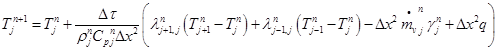(33)

For j=JZ, the difference equation can be obtained from the B.C. in Eq. 21, and the schematic diagram is shown in Fig. 8c.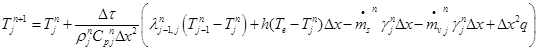(34)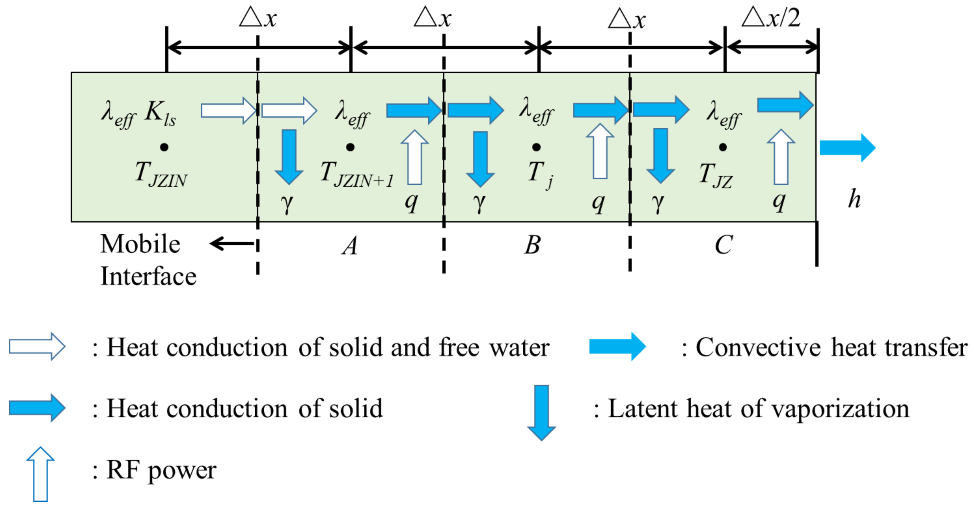Fig. 8. Difference equation of heat transfer below FSP

RESULTS AND DISCUSSION

Calculated Results

Total drying time and internal temperature are the most important parameters in industrial drying; however, the other parameters are also important because they affect the numerical value of MC and temperature in the calculation procedure. In this paper, the drying conditions were as follows: the initial MC was 1 (100%), the initial temperature of the wood was 25 C, the ambient temperature of the kiln was 25 C, and the ambient pressure was 20 KPa. The temperature of the center of the wood was controlled at 70 °C. The convective mass transfer coefficient was 0.04 m/s, the convective heat transfer coefficient was 10 W/m2·K, the RF power density was 6500W/m3, and the average vapor intrinsic permeability was 0.5 Darcy. The other parameters employed in the governing equations are listed in the appendix. The specimen was divided into 10 units along the direction of length (x axis), as is shown in Fig. 3. The time step was 1 s, and the frequency of recording of data was one time per 60 s. The distribution curves of some adjacent units of the independent variables were nearly the same; thus not all the distribution curves are depicted. The evolution curves of the main independent variables are shown as follows:

Figure 9 depicts the evolution curve of each cross-sectional average MC along the length direction. It indicates that the units closer to the end of the wood dried at a faster rate. The total drying process can be divided into three stages: (1) The whole pieces of wood were in the wet zone, and the MC of the unit j=10 (the end) reached FSP after about 29 h. In the initial drying period, the drying rate of the units closer to the center was lower than that of the units closer to the end; then the drying rate begin to speed up at about 10 h, especially for units j=1 and j=3; (2) From 29 to 42 h, the dry zone (the mobile interface) traveled from the end of the wood to the center of the wood, where the MC of the unit j=1 (the center) reached FSP. The drying rate of the unit in the dry zone was significantly slower than that of the unit in the wet zone, because there was no binding energy between the free water in the cell cavity and the cell wall. As the MC decreased, the binding energy increased; (3) The whole wood was in the dry zone, and the drying rate gradually decreased, until the drying process finally stopped when the MC reached the equilibrium MC. These results are in alignment with the experimental data.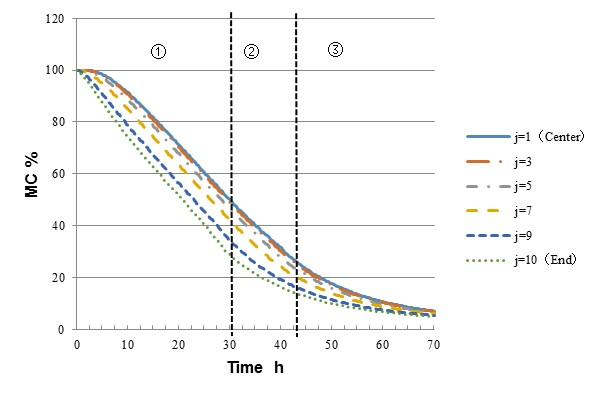Fig. 9. Evolution curve of MC

Figure 10 depicts the evolution curve of each cross-sectional average temperature along the direction of length. As can be seen, the units closer to the end of the wood had lower temperatures than the units near the center, and the temperature in the center was the highest. This is because the units near the end of the wood had lower a MC, which results in less RF power being absorbed by polar moisture molecules. Meanwhile, the ends of the wood were exchanging heat with the environment. The whole drying process can be divided into 3 stages: (1) The rapid heating of RF raised the temperature of the wood until the temperature of the unit j=1 reached the preset value 70 ˚C at about 10 h; (2) The temperature of part units was kept about constant with no obvious change, but the temperature of units j=10 and j=9 tended to decrease slightly, perhaps because the energy consumed by evaporation was increasing with the increasing amounts of free water evaporation in these two units; and (3) The entirety of the wood entered the dry zone, and the temperature of the units closer to the end of the wood gradually increased, because the volume evaporation rate decreased greatly with decreasing MC, and the energy consumed by evaporation was gradually reduced.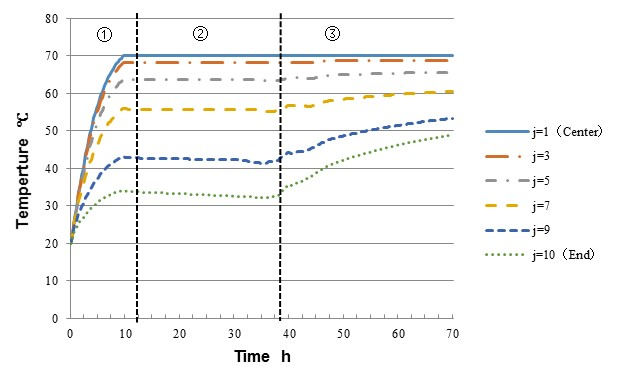Fig. 10. Evolution curve of temperature

Figure (11) depicts the evolution curve of each cross-sectional average vapor pressure along the direction of length. As stated above, the vapor pressure is determined by the temperature above FSP; it increased rapidly at the initial period and was kept about constant at the middle period, so the evolution curve of vapor pressure was the same as that of the temperature above FSP. The vapor was unsaturated during the later period of the drying process; therefore the changing trend of the vapor pressure and vapor density was not the same as that of the temperature. The vapor pressure decreased markedly with the transfer of vapor out of the wood in the form of bulk flow.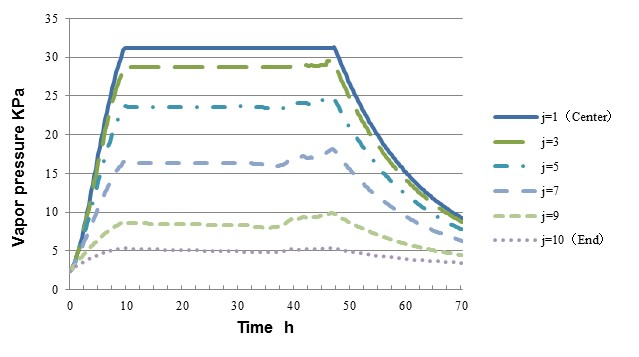Fig. 11. Evolution curve of vapor pressure

Figure (12) depicts the evolution curve of each cross-sectional average volume evaporation rate along the direction of length and surface evaporation rate. It indicates that the changing trend of the two variables was similar. The volume evaporation rate increased with increasing temperature at the beginning of drying, and tended to be kept about constant because of the continuous evaporation of free water. Finally, it decreased with decreasing MC because the amount of water was gradually reduced.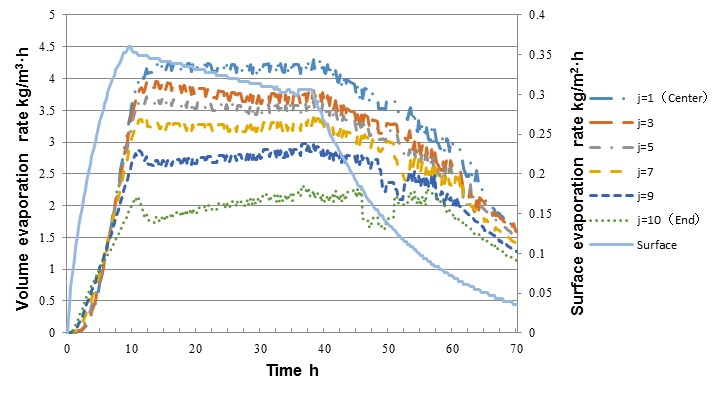Fig. 12. Evolution curve of volume evaporation rate and surface evaporation rate

Model Validation

In order to verify the accuracy of the model, a drying experiment with the same drying conditions as the model above was carried out. A 38-year-old log of Sugi wood from the Ehime University forest was used for specimen preparation with a basic density of 421 kg/m3 and the initial moisture of about 100%. The log was processed into lumber with the pith at the center and a size of 120 mm (width) × 120 mm (thickness) × 1000 mm (length). One end of the specimen was sealed with epoxide resin and all four sides were sealed with silicon resin to ensure that the mass and heat could only be transferred along the direction of length (x axis). The RF/V drying device is shown in Fig. 13, the fluctuating frequency of the RF generator was 27.12 MHz, and the effective output power was 1 kW. The other drying conditions were the same as those mentioned above.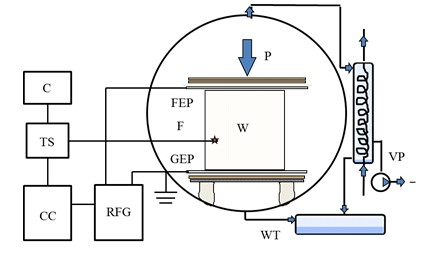Fig. 13. RF/V drying device; CC=Control cabinet; RFG=RF Generator; FEP=Power plate; GEP=Grounding plate; VP=Vacuum pump; C=Computer; TS=Temperature measurement system; F=Fiber optic sensor; W=Wood; WT=Water storage tank

Measurement positions of temperature and moisture content of the internal wood during the drying process are shown in Fig. 14. The notations ①,…⑦ represent the sections for temperature measurement;Ⅰ,…Ⅴ represent the sections for moisture content measurement. The temperature measurement positions are denoted by three numbers: the first number shows the position in the longitudinal direction; the second shows the location along the thickness direction (direction of power flow); and the third shows the location along the direction of width. The temperature was controlled at position 2.3.3, the center of cross section ②. The temperature was measured with two devices: ★ represents the fiber optic sensor (F); and ● represents the platinum resistance thermometer (Pt).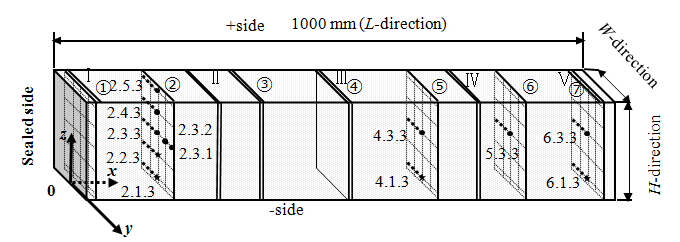Fig. 14. Positions for temperature and MC measurement

The comparison of experimental results (average MC and temperature of the wood) and calculated results is shown in Fig. 15. There was a strong consistency between the experimentally measured temperature and the calculated temperature: both increased rapidly (0 h to 12 h) until reaching the perset value at the initial period, then remained about constant until the end of the drying process (12 h to 70 h).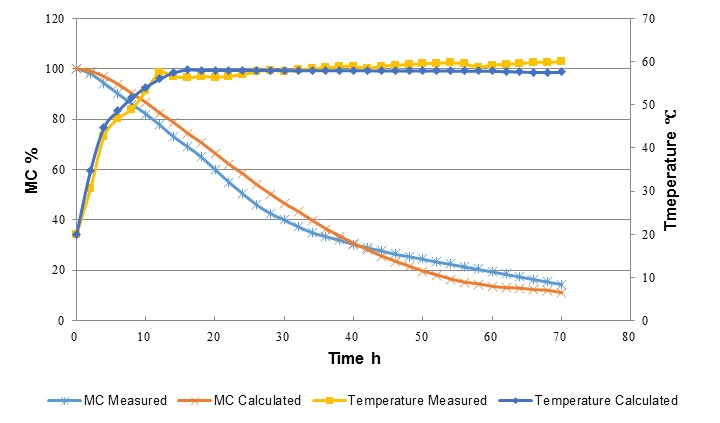Fig. 15. Comparison of experimental results and calculated results

The changing trend of calculated MC was similar to that of the experimentally measured MC, with a faster drying rate in the early stage than in the later stage. It can also be seen that the simulation precision of the temperature change was higher than that of MC, perhaps because of the following reasons: (1) The temperature was measured by fiber sensors, whose precision is higher, whereas the MC was measured by the weighing method, which has more room for error; (2) There exists diffusion in an actual drying process; thus above FSP, from 0 h to 40 h, the drying rate of experimentally measured MC was higher than that of calculated MC; and (3) The permeability of the wood decreased with decreasing MC; thus the drying rate of experimentally measured MC decreased from 40 h to 70 h. This model was in close agreement with the experimental results for temperature and moisture in the wood, and the square of the relevant coefficient of the calculated and experimental values for wood temperature and MC are 0.9914 and 0.9875, respectively, which indicates that the mathematical model could describe and predict the RF/V drying process of wood.

CONCLUSIONS

1. A one-dimensional mathematical model during the continuous RF/V drying of square-edged timber was developed and solved based on the heat and mass transfer theory of porous materials, while taking the characteristics of RF/V drying into account.
2. The change laws of the calculated results of the independent variable including MC, temperature, vapor pressure, etc., were analyzed.
3. By comparing the calculated results with the experimental results, it can be concluded that though the calculated results were not completely the same as the experimental results, their agreement with the experimental results was reasonable. Therefore, most of the important phenomena observed during RF/V drying were adequately described by this model.

ACKNOWLEDGMENTS

This project was supported by the National Natural Science Foundation of China, Grant No. 31270595 and 30972306.

REFERENCES CITED

Avranmidis, S., and Liu, F. (1994). “Drying characteristics of thick lumber in a laboratory radio-frequency/vacuum dryer,” Drying Technology 12(8), 1963-1981. DOI: 10.1080/07373939408962215

Cai, Y. C., and Hayashi, K. (2001a). “Pressure and temperature distribution in wood during radio frequency vacuum drying,” 7thInternational IUFRO Wood Drying Conference, 386-391.

Cai, Y. C., and Hayashi , K. (2001b). “Three-dimensional measurement of temperature distribution in wood during radio frequency vacuum drying,” Mokuzai Gakkaishi 47(5), 389-396.

Cai, Y. C., and Hayashi , K. (2002). “Contribution of evaporation from transverse sections to drying rate during radio-frequency/vacuum drying,” Mokuzai Gakkaishi. 48(2), 73-79.

Chen, P., and Schmidt, P. S. (1990). “An integral model for drying of hygroscopic and nonhygroscopic materials with dielectric heating,” Drying Technology 8(5), 907-930. DOI: 10.1080/07373939008959928

Chen, P., and Schmidt, P. S. (1997). “Mathematical modeling of dielectrically-enhanced

drying,” in: Mathematical Modeling and Numerical Techniques in Drying

Technology, I. Turner and A. S. Mujumdar, (eds.), Marcel Dekker, New York, 83-156.

Constant, T., Moyne, C., and Perre, P. (1996). “Drying with internal heat generation: Theoretical aspects and application to microwave heating,” AIChE J. 42(2), 359-368. DOI: 10.1002/aic.690420206

Couture, F., Fabrie, P., and Puiggali, J. R. (1995). “An alternative choice for the drying variables leading to a mathematically and physically well described problem,” Drying Technology 13(3), 519-550. DOI: 10.1080/07373939508916973

Defo, M., Fortin, Y., and Coutier, A. (1999). “Determination of the effective water conductivity of sugar maple sapwood and white spruce heartwood under vacuum,” Wood Fiber Sci. 31(4), 343-359.

Kamke, F. A., and Vanek, M. (1992). “Critical review of wood drying models: Plan of study,” Holzforschung and Holzverwertung 6, 81-83.

Kanagawa, Y. (1989). “Resin distribution in lumber dried by vacuum drying

combined with radio frequency,” in: Proceedings of 1st IUFRO Wood Drying

Conference, Seattle, WA, 158-163.

Kounmoutsakos, A., and Avranmidis, S. (1989). “Evaluation of “three-variable” models for the prediction of equilibrium moisture content in wood,” Wood Sci. Technol. 23(3), 251-257. DOI: 10.1007/BF00367738

Kounmoutsakos, A., Avranmidis, S., and Hatzikiriakos, S. G. (2001a). “Radio frequency vacuum drying of wood: I. Mathematical model,” Drying Technology 19(1), 65-84. DOI: 10.1081/DRT-100001352

Kounmoutsakos, A., Avranmidis, S., and Hatzikiriakos, S. G. (2001b). “Radio frequency vacuum drying of wood: II. Experimental model evaluation,” Drying Technology 19(1), 85-98. DOI: 10.1081/DRT-100001353

Li, X. J., Zhang, B. G., and Li W. J. (2008). “Microwave-vacuum drying of wood: Model formulation and verification,” Drying Technology 26(11), 1382-1387. DOI: 10.1080/07373930802333551

Perre, P. (1999). “How to get a relevant material model for wood drying simulation,” in: Proceedings of the 1st Wood Drying Workshop, Scotland, UK, 58-84.

Perre, P., and Turner, I. (1997). “The use of macroscopic equations to simulate heat and mass transfer in porous media: Some possibilities illustrated by a wide range of configurations that emphasize the role of internal pressure,” in: Mathematical Modeling and Numerical Techniques in Drying Technology, I. Turner and A. S. Mujumdar (eds.), Marcel Dekker, New York, pp. 83-156.

Rosen, N. H. (1987). “Recent advances in the drying of solid wood,” in: Advances in Drying, Vol. 4, A. S. Mujumdar (ed.), Hemisphere Publishing Corp., Bristol, PA, 99-146.

Siau, J. F. (1984). Transport Processes in Wood, Springer-Verlag, New York. DOI: 10.1007/978-3-642-69213-0

Siau, J. F. (1995). Wood: Influence of moisture on physical properties, VPI&SU Press,

Blacksburg, VA, 126.

Turner, I. W., and Jolly, P. G. (1991). “Combined microwave and convective drying of a porous material,” Drying Technology 9(5), 1209-1269. DOI: 10.1080/07373939108916749

Waananen, K. W., Lirchfield, J. B., and Okos, M. R. (1993). “Classification of drying models for porous solids,” Drying Technology 11(1), 1-40. DOI: 10.1080/07373939308916801

Wang, Y. Q. (2012). Mathematical model of flow and contaminant transport in porous media, Science Press, Beijing.

Yu, C. M. (2010). Numerical Analysis of Heat and Mass Transfer for Porous Materials, Tsinghua University Press, Beijing.

Zhou, B., and Avramidis, S. (1999). “On the loss factor of wood during radio frequency heating,” Wood Sci. Technol. 33, 299-310. DOI: 10.1007/s002260050117

Article submitted: January 16, 2015; Peer review completed: June 28, 2015; Revised version received: July 6, 2015; Accepted: July 7, 2015; Published: July 16, 2015.

DOI: 10.15376/biores.10.3.5440-5459